## The California sea lion is capable of making extremely fast, tight turns while swimming underwater. In one study, scientists observed a sea

Question

The California sea lion is capable of making extremely fast, tight turns while swimming underwater. In one study, scientists observed a sea lion making a circular turn with a radius of 0.37 m while swimming at 4.0 m/s.

What is the sea lion’s centripetal acceleration, in units of g?

What percentage is this acceleration of that of an F-15 fighter jet’s maximum centripetal acceleration of 9g?

in progress 0
5 months 2021-08-10T14:44:10+00:00 1 Answers 39 views 0

Acceleration of Sea Lion is 4.41 g

This is 49% of maximum jet acceleration given as a = 9g

Explanation:

As we know that the radius of the circular loop is given as

R = 0.37 m

The speed of the fish is given as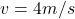Now the centripetal acceleration of the sea lion is given as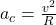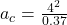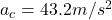as we know that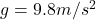so we have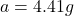Now Percentage of this acceleration wrt maximum jet acceleration is given as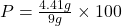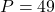%